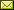## Learning Probabilities: Towards a Logic of Statistical Learning

 Alexandru Baltag Soroush Rafiee Rad Sonja Smets

 We propose a new model for forming beliefs and learning about unknown probabilities (such as the probability of picking a red marble from a bag with an unknown distribution of coloured marbles). The most widespread model for such situations of 'radical uncertainty' is in terms of imprecise probabilities, i.e. representing the agent's knowledge as a set of probability measures. We add to this model a plausibility map, associating to each measure a plausibility number, as a way to go beyond what is known with certainty and represent the agent's beliefs about probability. There are a number of standard examples: Shannon Entropy, Centre of Mass etc. We then consider learning of two types of information: (1) learning by repeated sampling from the unknown distribution (e.g. picking marbles from the bag); and (2) learning higher-order information about the distribution (in the shape of linear inequalities, e.g. we are told there are more red marbles than green marbles). The first changes only the plausibility map (via a 'plausibilistic' version of Bayes' Rule), but leaves the given set of measures unchanged; the second shrinks the set of measures, without changing their plausibility. Beliefs are defined as in Belief Revision Theory, in terms of truth in the most plausible worlds. But our belief change does not comply with standard AGM axioms, since the revision induced by (1) is of a non-AGM type. This is essential, as it allows our agents to learn the true probability: we prove that the beliefs obtained by repeated sampling converge almost surely to the correct belief (in the true probability). We end by sketching the contours of a dynamic doxastic logic for statistical learning.

In Lawrence S. Moss: Proceedings Seventeenth Conference on Theoretical Aspects of Rationality and Knowledge (TARK 2019), Toulouse, France, 17-19 July 2019, Electronic Proceedings in Theoretical Computer Science 297, pp. 35–49.
Published: 19th July 2019.

 ArXived at: https://dx.doi.org/10.4204/EPTCS.297.3 bibtex PDF
References in reconstructed bibtex, XML and HTML format (approximated).
 Comments and questions to:eptcs@eptcs.org For website issues:webmaster@eptcs.org